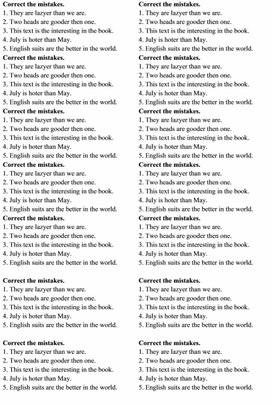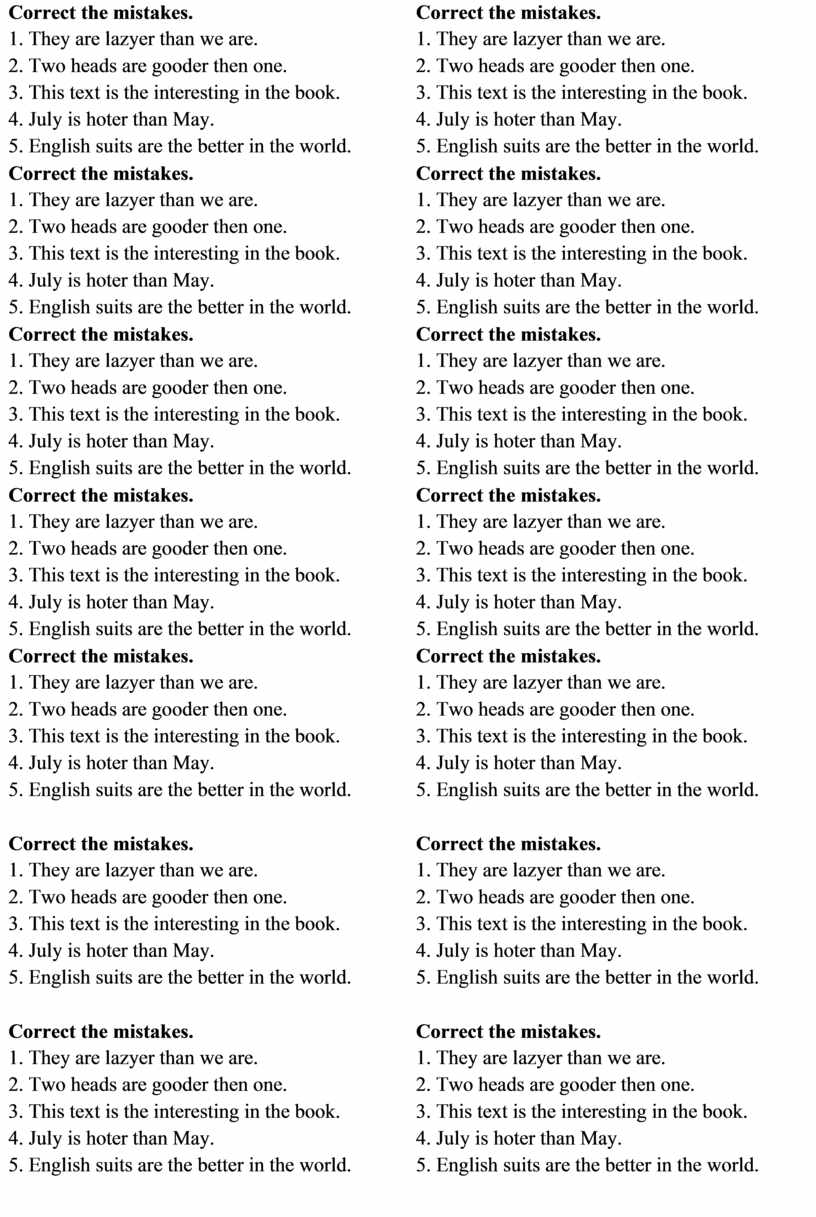Самостоятельная работа по английскому языку по теме "Степени сравнения прилагательных"
Оценка 4.7

# Самостоятельная работа по английскому языку по теме "Степени сравнения прилагательных"

Оценка 4.7
Контроль знаний
docx
английский язык
3 кл—5 кл
16.07.2022самостоятельная работа по английскому языку
Correct the mistakes.docx
 Correct the mistakes. 1. They are lazyer than we are. 2. Two heads are gooder then one. 3. This text is the interesting in the book. 4. July is hoter than May. 5. English suits are the better in the world. Correct the mistakes. 1. They are lazyer than we are. 2. Two heads are gooder then one. 3. This text is the interesting in the book. 4. July is hoter than May. 5. English suits are the better in the world. Correct the mistakes. 1. They are lazyer than we are. 2. Two heads are gooder then one. 3. This text is the interesting in the book. 4. July is hoter than May. 5. English suits are the better in the world. Correct the mistakes. 1. They are lazyer than we are. 2. Two heads are gooder then one. 3. This text is the interesting in the book. 4. July is hoter than May. 5. English suits are the better in the world. Correct the mistakes. 1. They are lazyer than we are. 2. Two heads are gooder then one. 3. This text is the interesting in the book. 4. July is hoter than May. 5. English suits are the better in the world. Correct the mistakes. 1. They are lazyer than we are. 2. Two heads are gooder then one. 3. This text is the interesting in the book. 4. July is hoter than May. 5. English suits are the better in the world. Correct the mistakes. 1. They are lazyer than we are. 2. Two heads are gooder then one. 3. This text is the interesting in the book. 4. July is hoter than May. 5. English suits are the better in the world. Correct the mistakes. 1. They are lazyer than we are. 2. Two heads are gooder then one. 3. This text is the interesting in the book. 4. July is hoter than May. 5. English suits are the better in the world. Correct the mistakes. 1. They are lazyer than we are. 2. Two heads are gooder then one. 3. This text is the interesting in the book. 4. July is hoter than May. 5. English suits are the better in the world. Correct the mistakes. 1. They are lazyer than we are. 2. Two heads are gooder then one. 3. This text is the interesting in the book. 4. July is hoter than May. 5. English suits are the better in the world. Correct the mistakes. 1. They are lazyer than we are. 2. Two heads are gooder then one. 3. This text is the interesting in the book. 4. July is hoter than May. 5. English suits are the better in the world. Correct the mistakes. 1. They are lazyer than we are. 2. Two heads are gooder then one. 3. This text is the interesting in the book. 4. July is hoter than May. 5. English suits are the better in the world. Correct the mistakes. 1. They are lazyer than we are. 2. Two heads are gooder then one. 3. This text is the interesting in the book. 4. July is hoter than May. 5. English suits are the better in the world. Correct the mistakes. 1. They are lazyer than we are. 2. Two heads are gooder then one. 3. This text is the interesting in the book. 4. July is hoter than May. 5. English suits are the better in the world.

## Correct the mistakes. 1. They are lazyer than we areМатериалы на данной страницы взяты из открытых истончиков либо размещены пользователем в соответствии с договором-офертой сайта. Вы можете сообщить о нарушении.